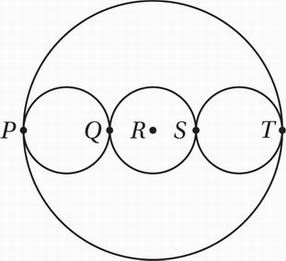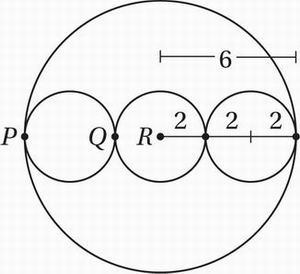# SAT Math Multiple Choice Question 632: Answer and Explanation

### Test Information

Question: 632

2.In the figure above, points P, Q, R, S, and T lie on the same line, and R is the center of the large circle. If the three smaller circles are congruent and the radius of the large circle is 6, what is the radius of one of the smaller circles?

• A. 1
• B. 2
• C. 3
• D. 4